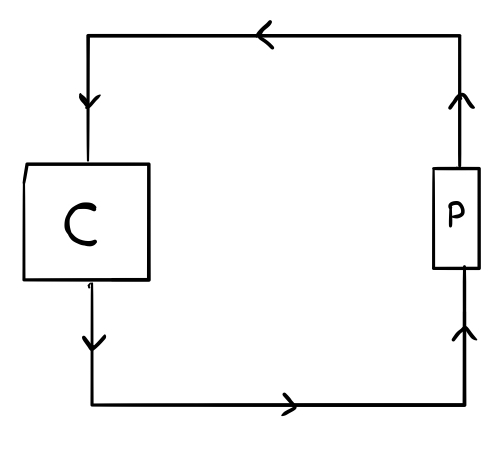## Predictors and Newcomb's Paradox

This post is about a concept from the philosophy of time and predetermination, namely the possibility of a device with the ability to perfectly predict the future. My philosophy club recently discussed this topic during a discussion about free will. One of my favorite authors, Ted Chiang, has also written a mind-bending short story (called “What’s Expected”) that uses the possibility of a “predictor” to explore the philosophy and psychology of free will.

First, let’s consider a thought experiment called “Newcomb’s Problem.” Imagine that you’re taking part in a game (similar to “Let’s Made a Deal!”) in which two boxes lie before you and each contains a certain amount of money. Box A is transparent and $\$10$can be seen inside. Box B is opaque, but you know that the game’s overseer has put either$\$100$ or $\$0$inside before the game began. You have to decide whether you want to take only Box B or both boxes (in other words, whether you want to be “greedy” or not). The game has just one more stipulation: the overseer can see the future, and the amount of money that he put in Box B depends on his prediction of your decision. If he predicted that you would be “greedy” (and take both boxes), then would have put no money in Box B, and otherwise he would fill it with$\$100$. How should one choose in order to maximize profit? Think about it for a moment, then consider the following two arguments, which come to different conclusions:

Argument B: If you take only Box B, then you’ll receive $\$100$, and otherwise you’ll only receive$\$10$, since the overseer will have foreseen your “greediness.” Therefore, take Box B.

Argument AB: Suppose that Box B contains $\$X$. If you take Box B, you’ll receive$\$X$, but if you take both boxes, they you’ll receive $\$X+\$10$. Obviously the second decision is better (in fact, exactly $\$10$better). Both arguments seem valid, even though they contradict each other. Which one is correct? In reality, it depends on which assumptions one takes. Let’s syllogistically lay out the assumptions of these two arguments. Argument B claims: • The overseer can perfectly predict the future. • Thus, you’ll receive$\$100$ if you take Box B and $\$10$if you take both boxes. • Therefore, you should take only Box B. And Argument B says: • The overseer has already placed a certain amount of money in Box B, and this amount can’t change. • In any case you’ll receive the contents of Box B, so you just have to choose whether you want an extra$\$10$ on top of that.
• If you want to optimize your winnings, you shouldn’t pass up free money, so take both boxes.

Now let’s place the assumptions of these two arguments next to each other:

• Argument B assumes that “the overseer can perfectly predict the future.”
• Argument AB assumes that “the overseer has already placed a certain amount of money in Box B, and this amount can’t change.”

Since the conclusions of Argument B and Argument AB are incompatible, the two assumptions can’t both be true. Thus, we come to the surprising conclusion that the existence of a perfect predictor entails that the contents of Box B can’t be determined and remains undetermined until you make your decision. This becomes problematic if we change the thought experiment a little bit: what would happen if Box B was transparent? If we believe in free will, obviously we couldn’t believe in perfect predictors - if one already knows a predictor’s prediction, then one can easily do the opposite.

However, this problem doesn’t depend on free will. Newcomb’s Paradox obfuscates a larger problem with predictors. Imagine that we had an electronic predictor-device that takes a signal as input and sends the same signal back one second earlier. Let’s put this device in a circuit like this:where C stands for a computer. Then we can program the computer with these instructions:

If a signal is received, do nothing for one second, and else if nothing is received for a few minutes, send a signal.

In this situation, a predictor could never work. When it predicts a signal, the computer stays quiet, and otherwise the computer sends out an impulse that the predictor hasn’t predicted. One might postulate that the computer would break down in this case, but that seems like a cop-out, since there’s no reason for the computer to break. Therefore we can conclude that the idea of a “perfect predictor” is unresolvably problematic, regardless of free will and determinism. Is there any hope of a solution to this problem, or are predictors totally impossible?